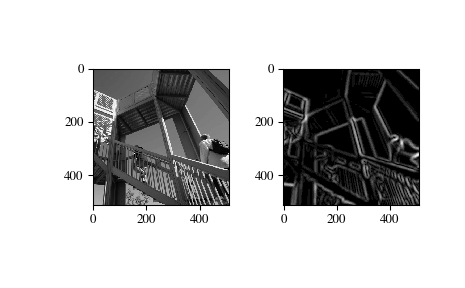scipy.ndimage.gaussian_gradient_magnitude(input, sigma, output=None, mode='reflect', cval=0.0, **kwargs)[source]

Multidimensional gradient magnitude using Gaussian derivatives.

Parameters: input : array_like The input array. sigma : scalar or sequence of scalars The standard deviations of the Gaussian filter are given for each axis as a sequence, or as a single number, in which case it is equal for all axes.. output : array or dtype, optional The array in which to place the output, or the dtype of the returned array. By default an array of the same dtype as input will be created. mode : str or sequence, optional The mode parameter determines how the input array is extended when the filter overlaps a border. By passing a sequence of modes with length equal to the number of dimensions of the input array, different modes can be specified along each axis. Default value is ‘reflect’. The valid values and their behavior is as follows: ‘reflect’ (d c b a | a b c d | d c b a) The input is extended by reflecting about the edge of the last pixel. ‘constant’ (k k k k | a b c d | k k k k) The input is extended by filling all values beyond the edge with the same constant value, defined by the cval parameter. ‘nearest’ (a a a a | a b c d | d d d d) The input is extended by replicating the last pixel. ‘mirror’ (d c b | a b c d | c b a) The input is extended by reflecting about the center of the last pixel. ‘wrap’ (a b c d | a b c d | a b c d) The input is extended by wrapping around to the opposite edge. cval : scalar, optional Value to fill past edges of input if mode is ‘constant’. Default is 0.0. Extra keyword arguments will be passed to gaussian_filter(). gaussian_gradient_magnitude : ndarray Filtered array. Has the same shape as input.

Examples

>>> from scipy import ndimage, misc
>>> import matplotlib.pyplot as plt
>>> fig = plt.figure()
>>> plt.gray()  # show the filtered result in grayscale
>>> ax1 = fig.add_subplot(121)  # left side
>>> ax2 = fig.add_subplot(122)  # right side
>>> ascent = misc.ascent()